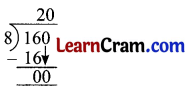# DAV Class 3 Maths Chapter 5 Worksheet 11 Solutions

The DAV Class 3 Maths Book Solutions and DAV Class 3 Maths Chapter 5 Worksheet 11 Solutions of Division offer comprehensive answers to textbook questions.

## DAV Class 3 Maths Ch 5 WS 11 Solutions

Question 1.
50 pencils are equally packed in 5 packets. How many pencils are there in each packet?
Solution:
No. of pencils in 5 packets = 50
No. of pencils in I packet = 50 ÷ 5 = 1010 pencils are there in 1 packet.Question 2.
Raju has 72 stamps. He pastes 8 stamps in each page of a scrap book. How many pages are used by Raju?
Solution:
72 stamps should be pasted
8 Stamps pasted = 1 page
1 Stamp pasted = $$\frac{1}{8}$$ page
72 Stamps pasted = $$\frac{1}{8}$$ × 72
= 72 ÷ 8 = 9 pages.
72 stamps pasted in 9 pages.

Question 3.
There are 35 biscuits in a packet, 5 girls want to share them equally. How many biscuits will each get?
Solution:
35 biscuits distributed among 5 girls
1 girl get = 35 ÷ 5 = 7Each girl gets 7 biscuits.Question 4.
Rahul can type 32 pages in 8 hours. How many pages can be typed in one hour9
Solution:
32 pages typed in 8 hours.
No. of pages typed in 1 hour = 32 ÷ 8 = 44 pages can be typed in one hour

Question 5.
60 marbles are equally shared among 10 boys. How many marbles will each boy get?
Solution:
10 boys shared No. of Marbles = 60
1 boy shared No. of Marbles = 60 ÷ 10 = 6
Each boy get 6 marbles.### DAV Class 3 Maths Chapter 5 Value Based Questions

Question 1.
In a colony, there was ……………….. garden was clean.

1. How many saplings did each girl plant?
Solution:
24 sapling

2. How do you care for your school garden?
Solution:
(a) By cleaning the garden. Picking up the fallen leaves.
(b) Watering the plants

3. Suggest other ways to keep the environment clean.
Solution:
(a) Put the garbage in garbage bin.
(b) Do not spit here and there. Make the people aware of problems cause by spitting here and there.
(c) Do not bum the leaves in garden or on road. In fact fill them in pit. This reduces the air pollution.

4. Which value is reflected by the children?
Solution:
They are environment conscious.### DAV Class 3 Maths Chapter 5 Worksheet 11 Notes

Question 1.
There are 160 mangoes in bucket. They are equally distributed among 8 persons. How many will each get?
Solution:
No. of mangoes 8 persons get = 160
No. of mangoes each person gets = 160 ÷ 8 = 20Each person get 20 mangoes.